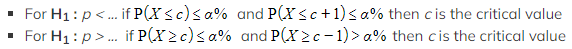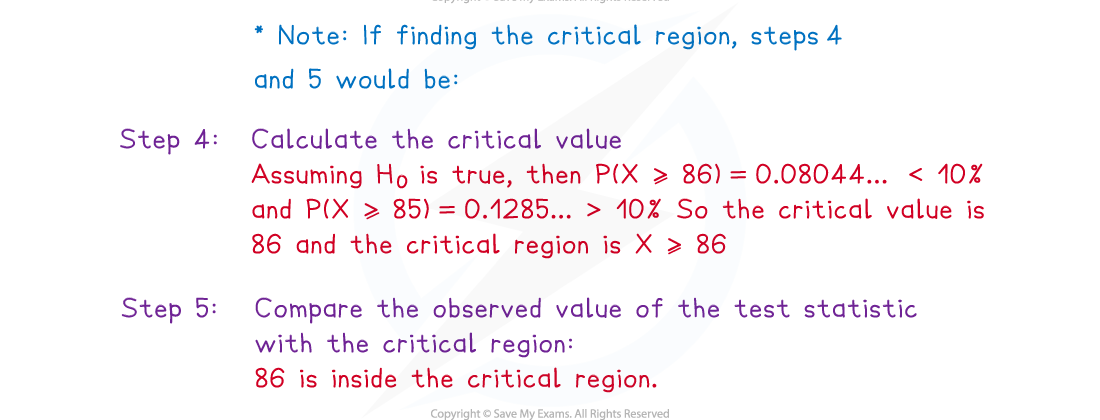# AQA A Level Maths: Statistics复习笔记5.2.1 Binomial Hypothesis Testing

### Binomial Hypothesis Testing

#### How is a hypothesis test carried out with the binomial distribution?

• The population parameter being tested will be the probability, p  in a binomial distribution B(n , p)
• A hypothesis test is used when the assumed probability is questioned
• The null hypothesis, H0  and alternative hypothesis, H1 will always be given in terms of p
• Make sure you clearly define p before writing the hypotheses
• The null hypothesis will always be H0 : p = ...
• The alternative hypothesis will depend on if it is a one-tailed or two-tailed test
• A one-tailed test would test to see if the value of p  has either increased or decreased
• The alternative hypothesis, H1 will be H1 : p > ...  or  H1 : p < ...
• A two-tailed test would test to see if the value of p  has changed
• The alternative hypothesis, H1 will be H1 : p ≠ ...
• To carry out a hypothesis test with the binomial distribution, the test statistic will be the number of successes in a defined number of trials
• When defining the test statistic, remember that the value of p  is being tested, so this should be written as p in the original definition, followed by the null hypothesis stating the assumed value of p
• The binomial distribution will be used to calculate the probability of the test statistic taking the observed value or a more extreme value
• The hypothesis test can be carried out by
• either calculating the probability of the test statistic taking the observed or a more extreme value (p – value) and comparing this with the significance level
• or by finding the critical region and seeing whether the observed value of the test statistic lies within it
• Finding the critical region can be more useful for considering more than one observed value or for further testing

#### How is the critical value found in a hypothesis test with the binomial distribution?

• The critical value will be the first value to fall within the critical region
• The binomial distribution is a discrete distribution so the probability of the observed value being within the critical region, given a true null hypothesis may be less than the significance level
• This is the actual significance level and is the probability of incorrectly rejecting the null hypothesis
• For a one-tailed test use your calculator to find the first value for which the probability of that or a more extreme value is less than the given significance level
• Check that the next value would cause this probability to be greater than the significance level
•For a two-tailed test you will need to find both critical values, one at each end of the distribution
• Use your calculator to find the first value for which the probability of that or a more extreme value is less than half of the given significance level in both the upper and lower tails
• Often one of the critical regions will be much bigger than the other

#### What steps should I follow when carrying out a hypothesis test with the binomial distribution?

• Step 1. Define the probability, p
• Step 2. Write the null and alternative hypotheses clearly using the form

H0 : p = ...

H1 : p = ...

•Step 4. Calculate either the critical value(s) or the p – value for the test
• Step 5. Compare the observed value of the test statistic with the critical value(s) or the p - value with the significance level
• Step 6. Decide whether there is enough evidence to reject H0 or whether it has to be accepted
• Step 7.Write a conclusion in context

#### Worked Example

Jacques, a breadmaker, claims that more than 80% of people that shop in a particular supermarket buy his brand of bread.  Jacques takes a random sample of 100 customers that have purchased bread and asks them which brand of bread they have purchased.  He records that 86 of them had purchased his brand of bread.  Test, at the 10% level of significance, whether Jacques’ claim is justified.#### Exam Tip

• Make sure you are careful with the inequalities when finding critical values from the binomial tables or from your calculator.